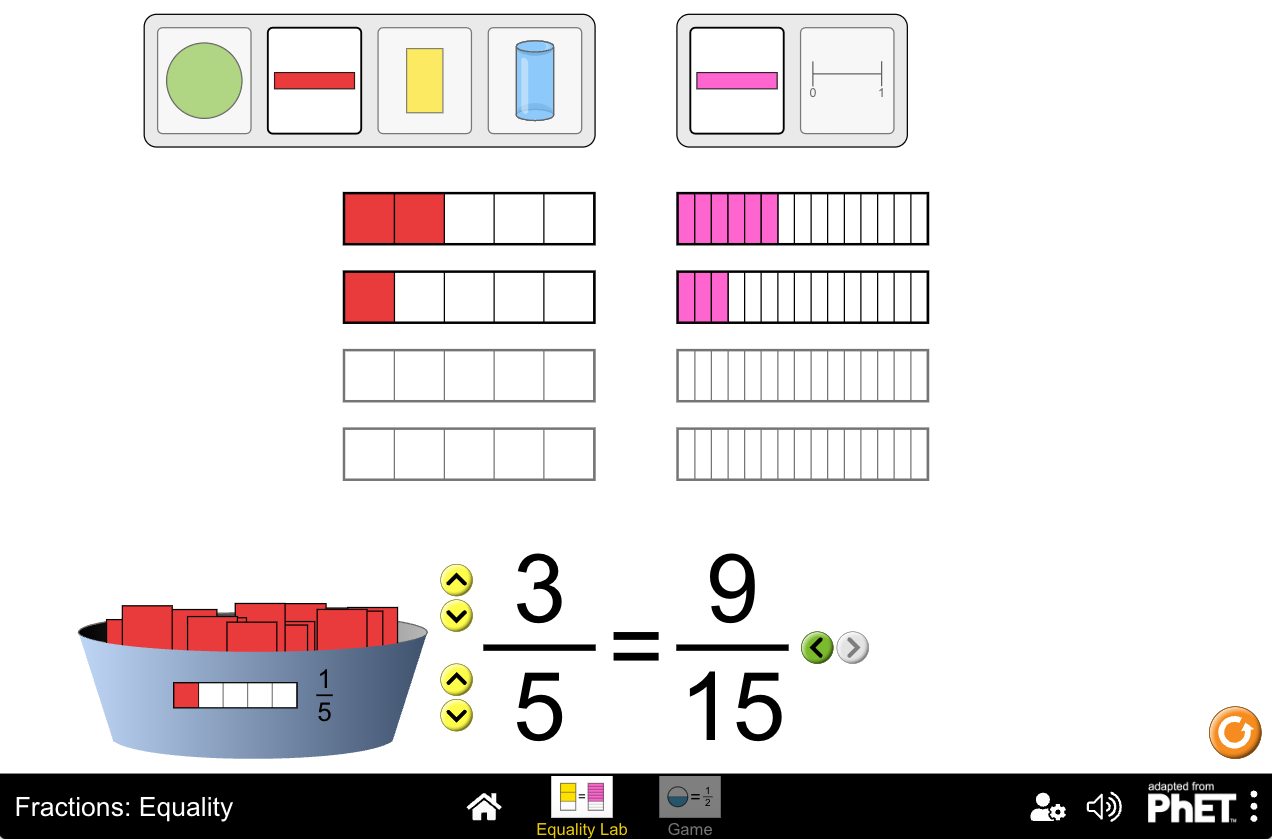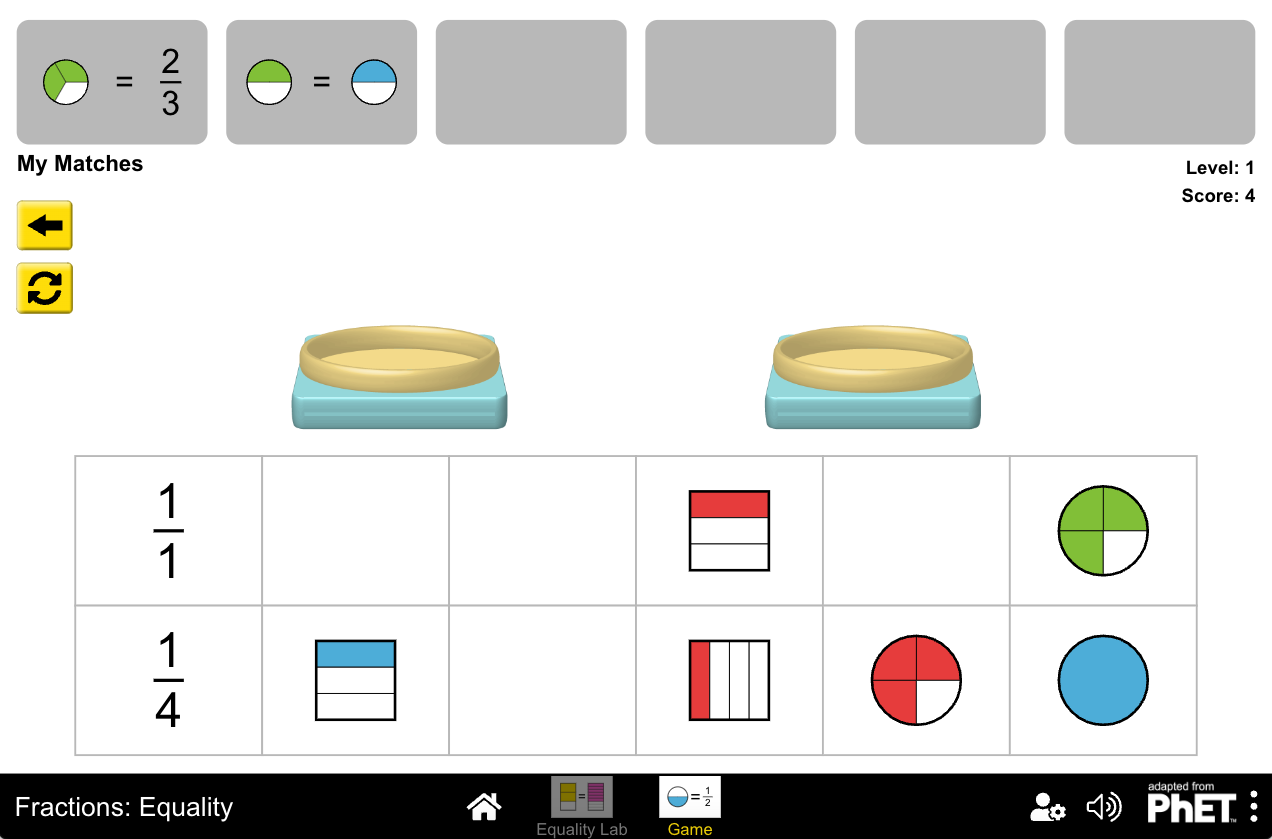MM Practice
×
Multiplication
Division
Place Value
Fractions & Decimals
Measurement
Statistics & Probability
Pre-Algebra
Money
Kindergarten
Geometry

# Equivalent Fractions with Visual ModelsAn interactive lab and matching game for grades 3-4

Explore equivalent fractions visually with this interactive online activity and game.

This activity includes two portions:

(1) Equality Lab which can be used for exploration and to illustrate the concept.

In the lab, the arrow buttons allow you to change the number of pieces and the kind of pieces (numerator and denominator) the fractions have. You can then drag fraction slices to the empty pies to make a fraction, and see an equivalent fraction on the other side of the equals sign.

You can choose between a rectangle, a circle, a cylinder, and a number line to visualize the fractions.

(2) A matching game, where you match visual fraction models with written fractions. The game has 8 levels of increasing difficulty, starting with simple fractions and advancing towards mixed numbers with larger numerators and denominator, such as 54/48.

The activity and game match the following Common Core math standards:

• 4.NF.A.1
Explain why a fraction a/b is equivalent to a fraction (n × a)/(n × b) by using visual fraction models, with attention to how the number and size of the parts differ even though the two fractions themselves are the same size. Use this principle to recognize and generate equivalent fractions.
• 3.NF.A.3b
Recognize and generate simple equivalent fractions, e.g., 1/2 = 2/4, 4/6 = 2/3. Explain why the fractions are equivalent, e.g., by using a visual fraction model.
• 3.NF.A.3a
Understand two fractions as equivalent (equal) if they are the same size, or the same point on a number line.

Credit: This activity is created by PhET

Screenshots from the game and activity:Allow my comment to be posted on this site